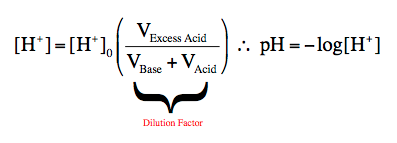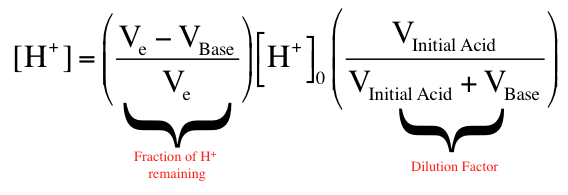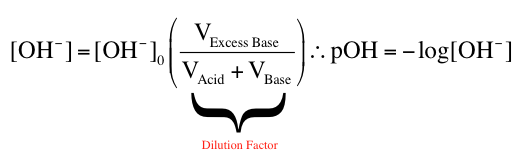Ch.10 - Acid-Base TitrationsWorksheetSee all chapters
 Ch.1 - Chemical Measurements 2hrs & 10mins 0% complete Worksheet Ch.2 - Tools of the Trade 1hr & 26mins 0% complete Worksheet Ch.3 - Experimental Error 1hr & 51mins 0% complete Worksheet Ch.4 + 5 - Statistics, Quality Assurance and Calibration Methods 2hrs & 1min 0% complete Worksheet Ch.6 - Chemical Equilibrium 3hrs & 43mins 0% complete Worksheet Ch.7 - Activity and the Systematic Treatment of Equilibrium 1hr & 1min 0% complete Worksheet Ch.8 - Monoprotic Acid-Base Equilibria 1hr & 52mins 0% complete Worksheet Ch.9 - Polyprotic Acid-Base Equilibria 2hrs & 16mins 0% complete Worksheet Ch.10 - Acid-Base Titrations 2hrs & 22mins 0% complete Worksheet Ch.11 - EDTA Titrations 1hr & 37mins 0% complete Worksheet Ch.12 - Advanced Topics in Equilibrium 1hr & 18mins 0% complete Worksheet Ch.13 - Fundamentals of Electrochemistry 2hrs & 27mins 0% complete Worksheet Ch.14 - Electrodes and Potentiometry 55mins 0% complete Worksheet Ch.15 - Redox Titrations 1hr & 14mins 0% complete Worksheet Ch.16 - Electroanalytical Techniques 1hr & 23mins 0% complete Worksheet Ch.17 - Fundamentals of Spectrophotometry 55mins 0% complete Worksheet BONUS: Chemical Kinetics Not available yet

# Strong Acid-Strong Base Titrations

See all sections

In a Strong Acid-Strong Base Titration we have the formation of a sigmodial shaped graph.

###### Strong Base-Strong Acid Titration

Concept #1: In these series of titrations the strong base is the starting solution or analyte and the strong acid is the titrant.

Concept #2: At all points before the equivalence point we calculate the amount of strong base remaining through a dilution factor equation.At the equivalence point for two strong species the pH will equal 7.00

Concept #3: After the equivalence point there will remain an excess of strong acid.Example #1: Calculate the pH of the solution resulting from the titration of 115.0 mL of 0.32 M KOH with 120.0 mL of 0.150 M HCl.

Practice: Calculate the pH of the solution resulting from the titration of 100.0 mL of 0.08 M LiH with 150.0 mL of 0.10 M Hl.

###### Strong Acid-Strong Base Titration

Concept #4: In these series of titrations the strong acid is the starting solution or analyte and the strong base is the titrant.

Concept #5: At all points before the equivalence point we calculate the amount of strong acid remaining through a dilution factor equation.Concept #6: After the equivalence point there will remain an excess of strong base.Example #2: Calculate the pH of the solution resulting from the titration of 250.0 mL of 0.370 M HBrO4 with 150.0 mL of 0.200 M NaH.

Example #3: Initially 7.1 g of HCl is titrated with 9.5 g of NaOH thereby making a solution that has a volume of 12.85 L. What is the pH of the solution?

a) 1.82

b) 2.48

c) 7.00

d) 11.52

e) 12.27